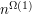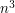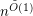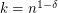## Пятница 25.09. Alexander Golovnev: "Polynomial Data Structure Lower Bounds in the Group Model"

Пятница, 25 сентября, Zoom. Начало в 18:00.

Докладчик: Alexander Golovnev (Georgetown University, USA).

Тема: Polynomial Data Structure Lower Bounds in the Group Model.

### Abstract

Proving super-logarithmic data structure lower bounds in the static group model has been a fundamental challenge in computational geometry since the early 80's. We prove a polynomial () lower bound for an explicit range counting problem ofconvex polygons in(each withfacets/semialgebraic-complexity), against linear storage arithmetic data structures in the group model. Our construction and analysis are based on a combination of techniques in Diophantine approximation, pseudorandomness, and compressed sensing---in particular, on the existence and partial derandomization of optimal \emph{binary} compressed sensing matrices in the polynomial sparsity regime (). As a byproduct, this establishes a (logarithmic) separation between compressed sensing matrices and the stronger RIP property.

The talk is based on the following paper: https://eccc.weizmann.ac.il/report/2020/057/